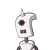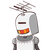# 23. A man borrows Rs 8,000 at a compound interest rate of 10% per annum. If he repays Rs 1,500 at theend of the first year a

23. A man borrows Rs 8,000 at a compound interest rate of 10% per annum. If he repays Rs 1,500 at the
end of the first year and Rs 2,000 at the end of the second year, find his outstanding loan at the
beginning of the third
year.​

### 2 thoughts on “23. A man borrows Rs 8,000 at a compound interest rate of 10% per annum. If he repays Rs 1,500 at the<br />end of the first year a”

1.explaination:

amount to be paid at the end of two years will be=

Amount after two years =

8000(1+10/100)square=8000(1.1)square

2.